# RS Aggarwal Solutions for Class 12 Maths Chapter 11: Applications of Derivatives Exercise 11D

Lagrange’s Mean-value Theorem and its significance are the topics which are discussed under this exercise. A huge number of solved examples are available before each exercise in order to make students familiar with the problems that would appear in the exam. Students who wish to score well in Mathematics can boost their exam preparation with the help of solutions designed by experts. RS Aggarwal Solutions Class 12 Maths Chapter 11 Applications of Derivatives Exercise 11D PDF links are given below.

## RS Aggarwal Solutions for Class 12 Maths Chapter 11: Applications of Derivatives Exercise 11D Download PDF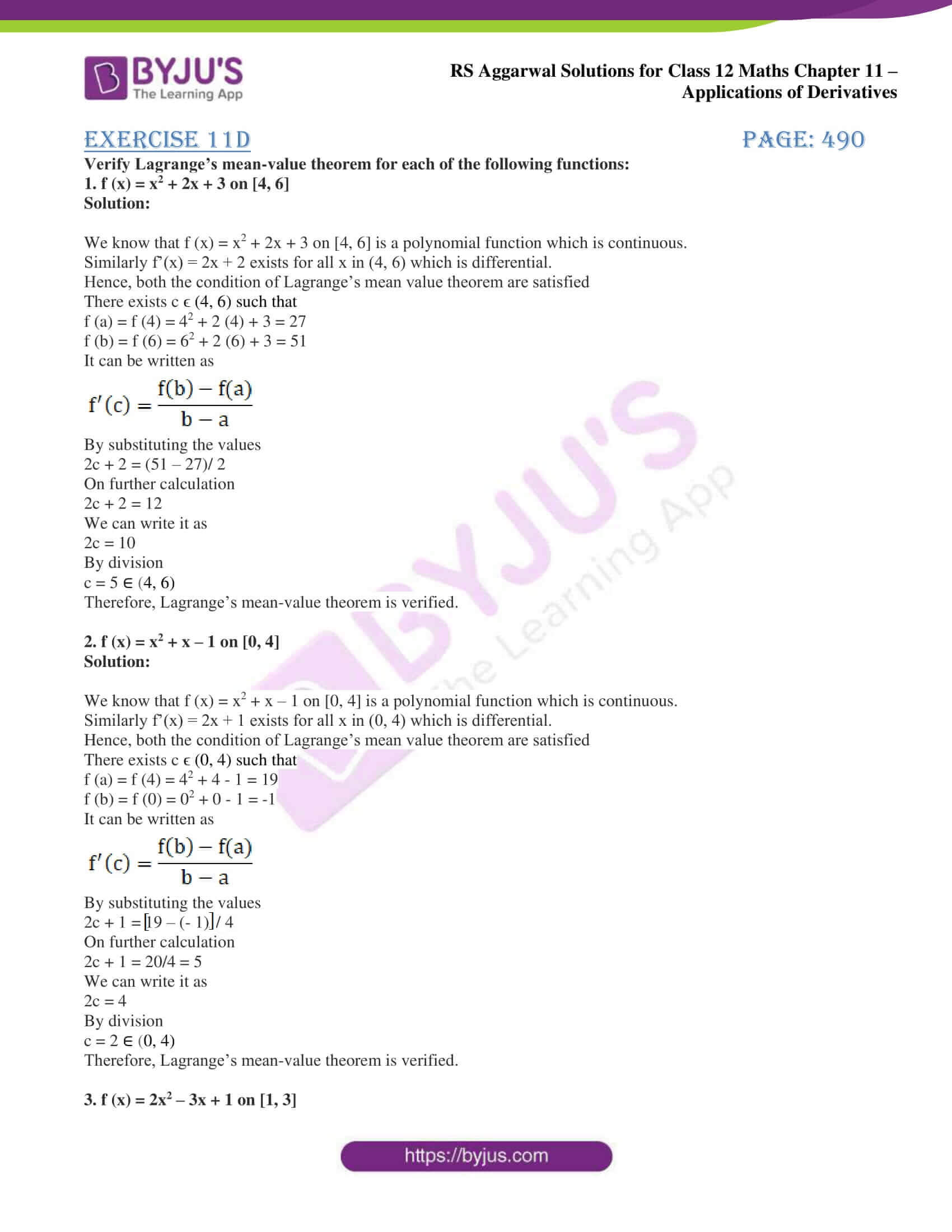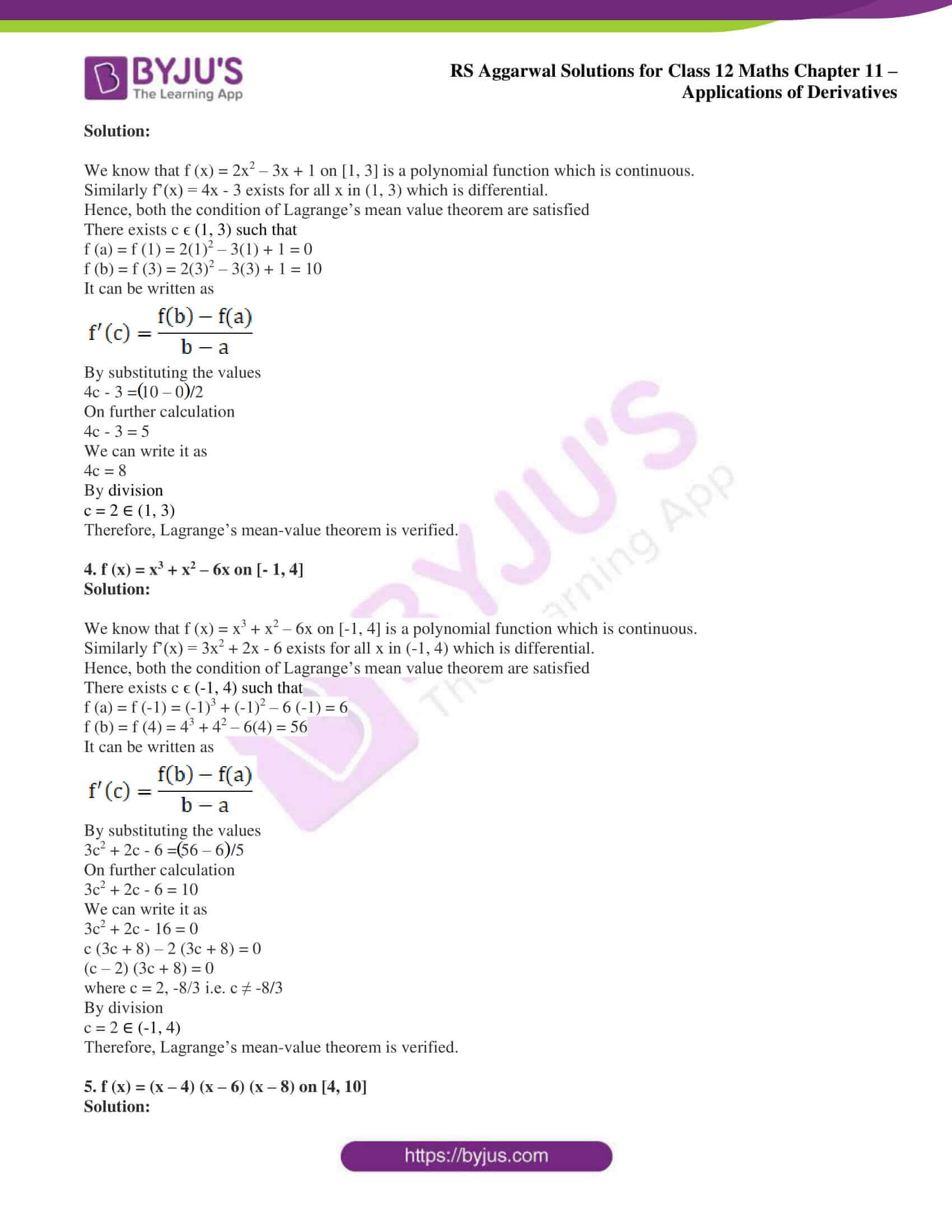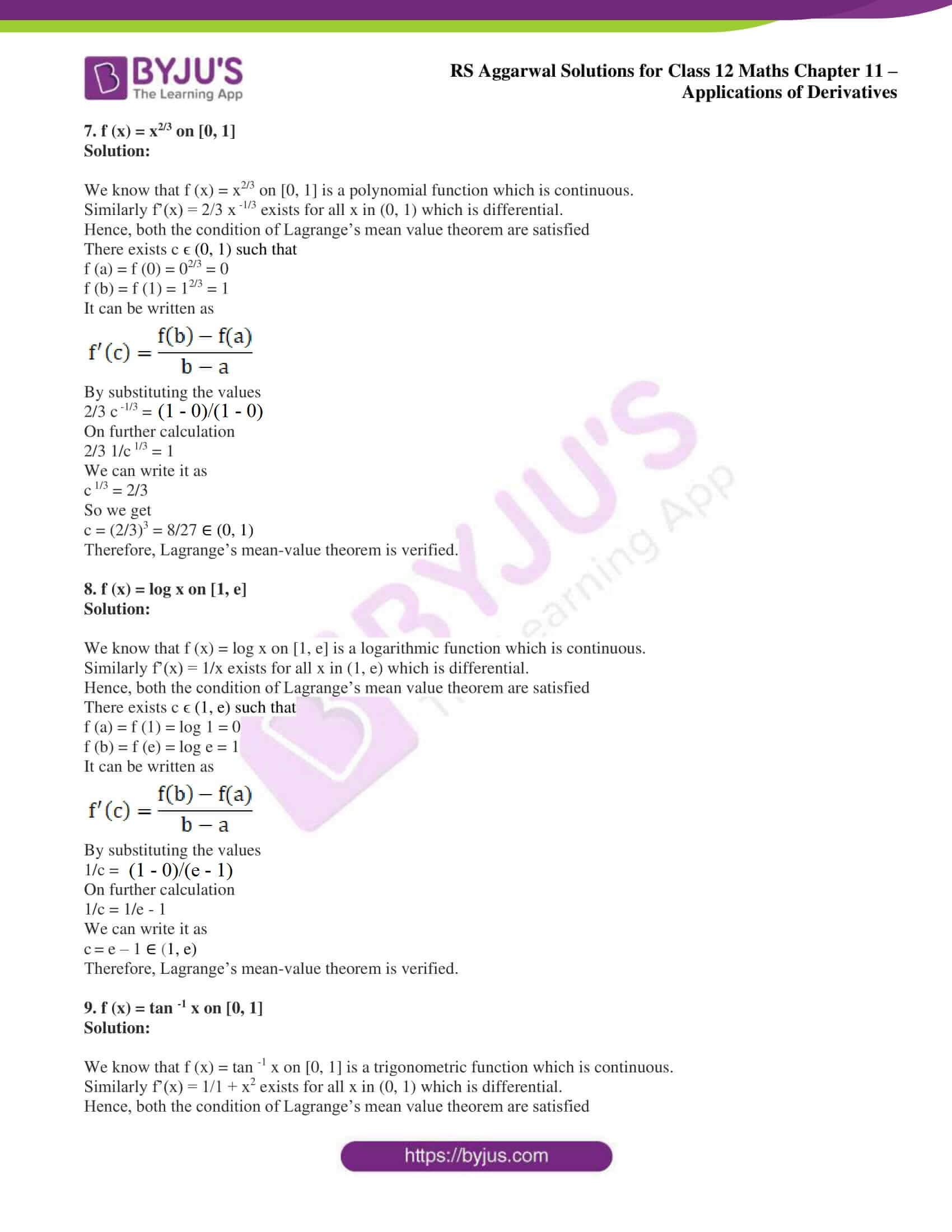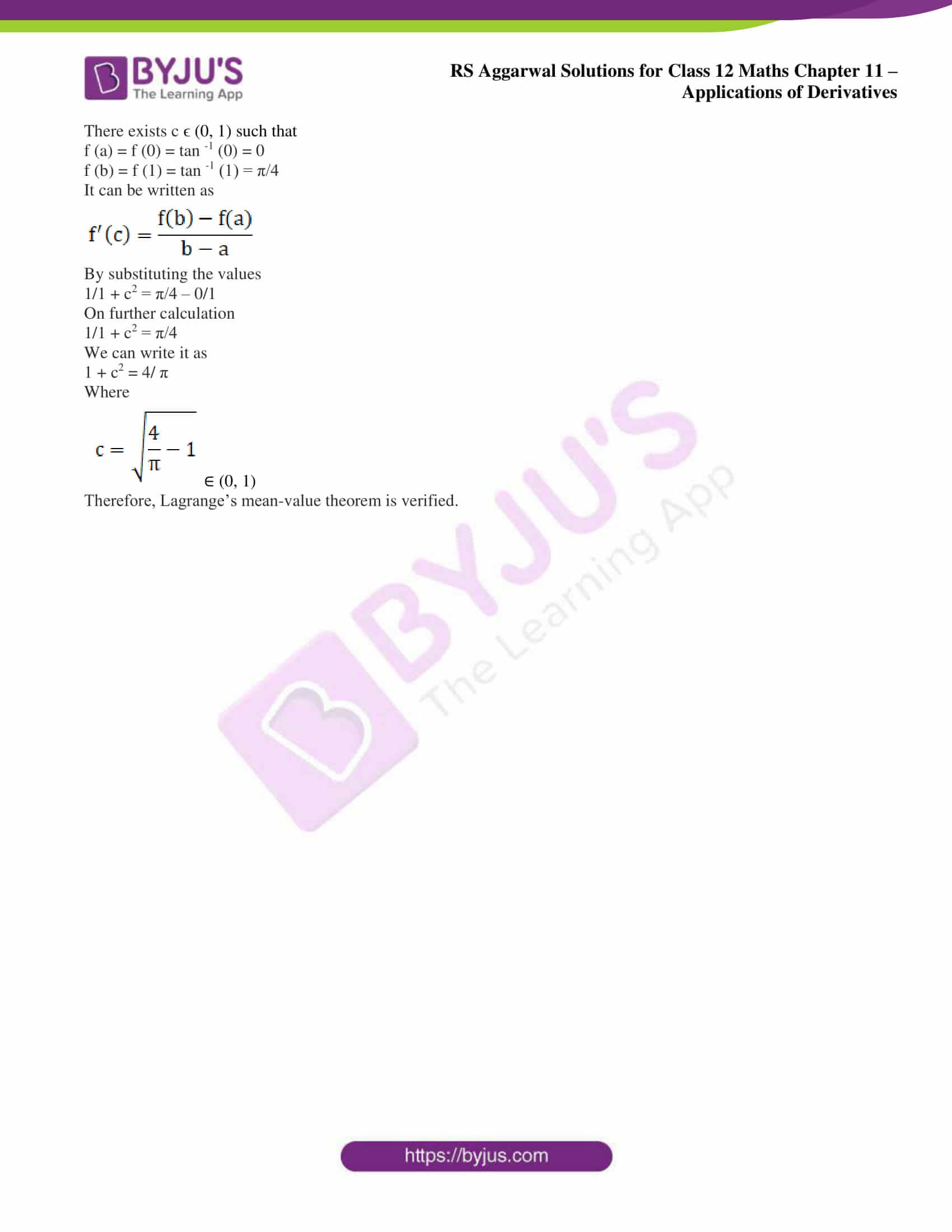### Access other exercise solutions of Class 12 Maths Chapter 11: Applications of Derivatives

Exercise 11A Solutions

Exercise 11B Solutions

Exercise 11C Solutions

Exercise 11E Solutions

Exercise 11F Solutions

Exercise 11G Solutions

Exercise 11H Solutions

### Access RS Aggarwal Solutions for Class 12 Maths Chapter 11: Applications of Derivatives Exercise 11D

Verify Lagrange’s mean-value theorem for each of the following functions:

1. f (x) = x2 + 2x + 3 on [4, 6]

Solution:

We know that f (x) = x2 + 2x + 3 on [4, 6] is a polynomial function which is continuous.

Similarly f’(x) = 2x + 2 exists for all x in (4, 6) which is differential.

Hence, both the condition of Lagrange’s mean value theorem are satisfied

There exists c ϵ (4, 6) such that

f (a) = f (4) = 42 + 2 (4) + 3 = 27

f (b) = f (6) = 62 + 2 (6) + 3 = 51

It can be written as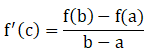By substituting the values

2c + 2 = (51 – 27)/ 2

On further calculation

2c + 2 = 12

We can write it as

2c = 10

By division

c = 5 ∈ (4, 6)

Therefore, Lagrange’s mean-value theorem is verified.

2. f (x) = x2 + x – 1 on [0, 4]

Solution:

We know that f (x) = x2 + x – 1 on [0, 4] is a polynomial function which is continuous.

Similarly f’(x) = 2x + 1 exists for all x in (0, 4) which is differential.

Hence, both the condition of Lagrange’s mean value theorem are satisfied

There exists c ϵ (0, 4) such that

f (a) = f (4) = 42 + 4 – 1 = 19

f (b) = f (0) = 02 + 0 – 1 = -1

It can be written asBy substituting the values

2c + 1 = [19 – (- 1)]/ 4

On further calculation

2c + 1 = 20/4 = 5

We can write it as

2c = 4

By division

c = 2 ∈ (0, 4)

Therefore, Lagrange’s mean-value theorem is verified.

3. f (x) = 2x2 – 3x + 1 on [1, 3]

Solution:

We know that f (x) = 2x2 – 3x + 1 on [1, 3] is a polynomial function which is continuous.

Similarly f’(x) = 4x – 3 exists for all x in (1, 3) which is differential.

Hence, both the condition of Lagrange’s mean value theorem are satisfied

There exists c ϵ (1, 3) such that

f (a) = f (1) = 2(1)2 – 3(1) + 1 = 0

f (b) = f (3) = 2(3)2 – 3(3) + 1 = 10

It can be written asBy substituting the values

4c – 3 = (10 – 0)/2

On further calculation

4c – 3 = 5

We can write it as

4c = 8

By division

c = 2 ∈ (1, 3)

Therefore, Lagrange’s mean-value theorem is verified.

4. f (x) = x3 + x2 – 6x on [- 1, 4]

Solution:

We know that f (x) = x3 + x2 – 6x on [-1, 4] is a polynomial function which is continuous.

Similarly f’(x) = 3x2 + 2x – 6 exists for all x in (-1, 4) which is differential.

Hence, both the condition of Lagrange’s mean value theorem are satisfied

There exists c ϵ (-1, 4) such that

f (a) = f (-1) = (-1)3 + (-1)2 – 6 (-1) = 6

f (b) = f (4) = 43 + 42 – 6(4) = 56

It can be written asBy substituting the values

3c2 + 2c – 6 = (56 – 6)/5

On further calculation

3c2 + 2c – 6 = 10

We can write it as

3c2 + 2c – 16 = 0

c (3c + 8) – 2 (3c + 8) = 0

(c – 2) (3c + 8) = 0

where c = 2, -8/3 i.e. c ≠ -8/3

By division

c = 2 ∈ (-1, 4)

Therefore, Lagrange’s mean-value theorem is verified.

5. f (x) = (x – 4) (x – 6) (x – 8) on [4, 10]

Solution:

We know that f (x) = (x – 4) (x – 6) (x – 8) can be written as f (x) = x3 – 18x2 + 104x – 192 on [4, 10] is a polynomial function which is continuous.

Similarly f’(x) = 3x2 – 36x + 104 exists for all x in (4, 10) which is differential.

Hence, both the condition of Lagrange’s mean value theorem are satisfied

There exists c ϵ (4, 10) such that

f (a) = f (4) = (4 – 4) (4 – 6) (4 – 8) = 0

f (b) = f (10) = (10 – 4) (10 – 6) (10 – 8) = 48

It can be written asBy substituting the values

3c2 – 36c + 104 = (48 – 0)/6

On further calculation

3c2 – 36c + 104 – 8 = 0

We can write it as

3c2 – 36c + 96 = 0

3 (c2 – 12c + 32) = 0

c2 – 12c + 32 = 0

So we get

c2 – 8c – 4c + 32 = 0

Taking c as common

c (c – 8) – 4 (c – 8) = 0

(c – 4) (c – 8) = 0

where c = 4, 8

By division

c = 8 ∈ (4, 10) and c = 4 ∉ (4, 10)

Therefore, Lagrange’s mean-value theorem is verified.

6. f (x) = ex on [0, 1]

Solution:

We know that f (x) = ex on [0, 1] is an exponential function which is continuous.

Similarly f’(x) = ex exists for all x in (0, 1) which is differential.

Hence, both the condition of Lagrange’s mean value theorem are satisfied

There exists c ϵ (0, 1) such that

f (a) = f (0) = e0 = 1

f (b) = f (1) = e1 = e

It can be written asBy substituting the values

ec = (e – 1)/ 1 – 0

So we get

ec = (e – 1)

Taking log on both sides

loge ec = loge (e – 1)

where c = loge (e – 1) ϵ (0, 1)

Therefore, Lagrange’s mean-value theorem is verified.

7. f (x) = x2/3 on [0, 1]

Solution:

We know that f (x) = x2/3 on [0, 1] is a polynomial function which is continuous.

Similarly f’(x) = 2/3 x -1/3 exists for all x in (0, 1) which is differential.

Hence, both the condition of Lagrange’s mean value theorem are satisfied

There exists c ϵ (0, 1) such that

f (a) = f (0) = 02/3 = 0

f (b) = f (1) = 12/3 = 1

It can be written asBy substituting the values

2/3 c -1/3 = (1 – 0)/(1 – 0)

On further calculation

2/3 1/c 1/3 = 1

We can write it as

c 1/3 = 2/3

So we get

c = (2/3)3 = 8/27 ∈ (0, 1)

Therefore, Lagrange’s mean-value theorem is verified.

8. f (x) = log x on [1, e]

Solution:

We know that f (x) = log x on [1, e] is a logarithmic function which is continuous.

Similarly f’(x) = 1/x exists for all x in (1, e) which is differential.

Hence, both the condition of Lagrange’s mean value theorem are satisfied

There exists c ϵ (1, e) such that

f (a) = f (1) = log 1 = 0

f (b) = f (e) = log e = 1

It can be written asBy substituting the values

1/c = (1 – 0)/(e – 1)

On further calculation

1/c = 1/e – 1

We can write it as

c = e – 1 ∈ (1, e)

Therefore, Lagrange’s mean-value theorem is verified.

9. f (x) = tan -1 x on [0, 1]

Solution:

We know that f (x) = tan -1 x on [0, 1] is a trigonometric function which is continuous.

Similarly f’(x) = 1/1 + x2 exists for all x in (0, 1) which is differential.

Hence, both the condition of Lagrange’s mean value theorem are satisfied

There exists c ϵ (0, 1) such that

f (a) = f (0) = tan -1 (0) = 0

f (b) = f (1) = tan -1 (1) = π/4

It can be written asBy substituting the values

1/1 + c2 = π/4 – 0/1

On further calculation

1/1 + c2 = π/4

We can write it as

1 + c2 = 4/ π

Where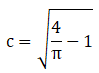∈ (0, 1)

Therefore, Lagrange’s mean-value theorem is verified.